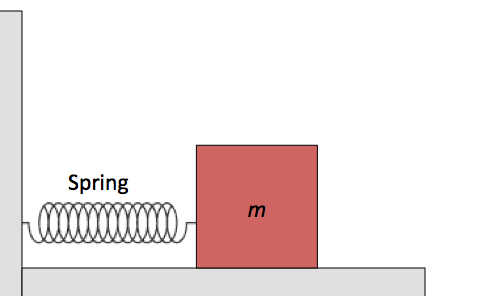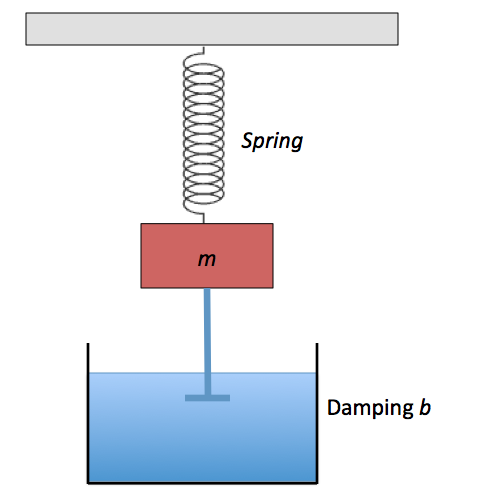Classical Mechanics

# Exponential decay from damping forcesIn the above diagram, a mass of $$m = 400 \text{ g}$$ is attached to a spring with spring constant $$k = 75 \text{ N/m}.$$ Since the floor is made of a special material, the friction between the floor and the mass exerts a damping force with damping constant $$b = 3 \text{ kg/s}$$ which is proportional to the velocity $$v$$ of the mass. What is the approximate ratio (in percentage terms) of the amplitude of the damped oscillations to the initial amplitude at the end of $$1$$ cycle?In the above diagram, a mass $$m (= 3 \text{ kg})$$ is attached to a spring with spring constant $$k = 51 \text{ N/m}.$$ A vane, attached to the mass $$m$$ and immersed in a liquid, exerts a damping force $$F_d$$ with damping constant $$b = 6 \text{ kg/s}$$ which is proportional to the velocity $$v$$ of the vane and mass. Find the approximate period of the motion.

Assume that the gravitational force is negligible relative to the damping force $$F_d$$ and the force exerted by the spring $$F_s.$$In the above diagram, a mass $$m (= 4 \text{ kg})$$ is attached to a spring with spring constant $$k = 20 \text{ N/m}.$$ A vane, attached to the mass $$m$$ and immersed in a liquid, exerts a damping force $$F_d$$ with damping constant $$b = 4 \text{ kg/s}$$ which is proportional to the velocity $$v$$ of the vane and mass. Approximately how long does it take for the amplitude of the damped oscillation to drop to half its initial value?

Assume that the gravitational force is negligible relative to the damping force $$F_d$$ and the force exerted by the spring $$F_s.$$In the above diagram, a mass $$m (= 5 \text{ kg})$$ is attached to a spring with spring constant $$k = 85 \text{ N/m}.$$ A vane, attached to the mass $$m$$ and immersed in a liquid, exerts a damping force $$F_d$$ with damping constant $$b = 3 \text{ kg/s}$$ which is proportional to the velocity $$v$$ of the vane and mass. Approximately how long does it take for the mechanical energy of the oscillator to drop to $$\frac{1}{3}$$ of its initial value?

Assume that the gravitational force is negligible relative to the damping force $$F_d$$ and the force exerted by the spring $$F_s.$$In the above diagram, a mass of $$m = 3 \text{ kg}$$ is attached to a spring with spring constant $$k = 15 \text{ N/m}.$$ Since the floor is made of a special material, the friction between the floor and the mass exerts a damping force with damping constant $$b$$ which is proportional to the velocity $$v$$ of the mass. If the mass is oscillating with time period $$T = 3.14 \text{ s},$$ what is the approximate damping constant?

×

Problem Loading...

Note Loading...

Set Loading...